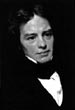Electrification - Math Matters - Maplesoft

# Electrification

Although various principles of electricity have been known for centuries, it is only since the formulation of key mathematical models that scientists and engineers have been able to fully harness this power.Maxwell’s equations: James Clerk Maxwell (Scottish, 1831 – 1879) developed a series of equations that described the fundamental laws of electricity and magnetism. His impact on the study of electromagnetics has been compared to Newton’s impact on mechanics. Maxwell’s rigorous application of mathematics became the new model for scientific communication.

Maxwell’s Equations in Differential form:Where:

D: displacement field

B: magnetic induction field

E: electric field

H: magnetic field

J: current density### Measures of greatness:

Farad (capacitance), Volt (voltage), Ohm (resistance), and Amp (current)Alessandro Volta
(Italian, 1745 – 1827)
pioneered the development of the electric battery.André-Marie Ampère
(French, 1775 – 1836)
was one of the main discoverers of electromagnetism.Georg Ohm
(German, 1789 – 1854)
developed Ohm’s Law, one of the basic laws of electricity and physics.Michael Faraday
(English, 1791-1867)
demonstrated that changing a magnetic field creates an electric field.